Author: Oscar Cronquist Article last updated on January 09, 2018

Today I want to share some pretty useful macros.

My first macro copies an excel defined table with vba. It is easy.

```Sub CopyTable()
Range("Table1[#All]").Copy Destination:=Worksheets("2010").Range("A13")
End Sub```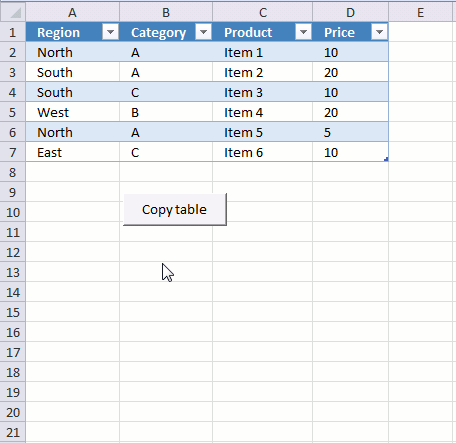It copies all table headers and data to sheet 2010, cell A13.

### Copy a filtered table

The macro above works great if there are no filters applied to the table. If there were filters applied, all data would have been copied anyway. So how do we solve that problem?

```Sub CopyFilteredTable()
Dim rng As Range
Dim WS As Worksheet
For Each Row In Range("Table2[#All]").Rows
If Row.EntireRow.Hidden = False Then
If rng Is Nothing Then Set rng = Row
Set rng = Union(Row, rng)
End If
Next Row
Set WS = Sheets.Add
rng.Copy Destination:=WS.Range("A1")
End Sub
```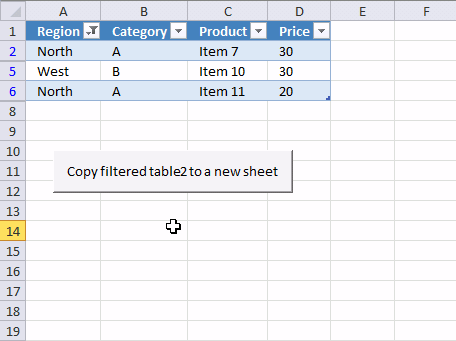This macro checks if each row in Table1 is visible. If it is, it is copied to a new sheet.

### Copy multiple filtered tables

The following macro cycles through each sheet in the current workbook and looks for excel tables. It copies all visible values from every excel table to a new sheet.

This is handy if you have many excel tables in a workbook and you want to merge all filtered values from all tables to a new sheet.

```Sub CopyFilteredTables()
Dim WS As Worksheet
Dim WSN As Worksheet
Dim tbl As ListObject
Dim rng As Range
Set WSN = Sheets.Add
For Each WS In Worksheets
For Each tbl In WS.ListObjects
Set rng = Nothing
For Each Row In tbl.DataBodyRange.Rows
If Row.EntireRow.Hidden = False Then
If rng Is Nothing Then Set rng = Row
Set rng = Union(Row, rng)
End If
Next Row
Lrow = WSN.Range("A" & Rows.Count).End(xlUp).Row
If Lrow > 1 Then Lrow = Lrow + 1
rng.Copy Destination:=WSN.Range("A" & Lrow)
Next tbl
Next WS
End Sub
```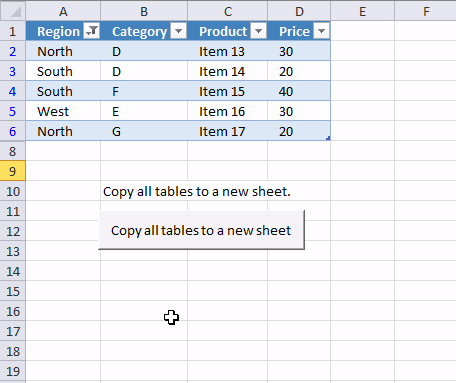### Apply filter to all excel tables in a workbook

Wouldn't it be great if you could apply the same filter to all excel tables in a workbook? This is useful if you are working with many excel tables but they all have the same table headers.

Check this macro out.

Instructions

1. Type the corresponding header, make sure you spell it right.
2. Type the critera below each header
3. Select the criteria with your mouse
4. Run macro ApplyFilterToTable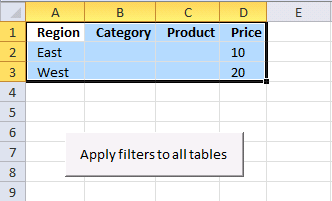```Sub ApplyFilterToTable()
Dim filters As Range
Dim flV() As Variant

Set fltrs = Selection

ReDim flV(0 To Selection.Rows.CountLarge - 2)

'Cycle through all sheets in active workbook
For Each WS In Worksheets
'Cycle through all excel tables in sheet
For Each tbl In WS.ListObjects
'Compare table headers to selection
For ct = 1 To tbl.DataBodyRange.Columns.Count
For cf = 1 To fltrs.Columns.Count
'Build array with filter values
j = 0
For i = LBound(flV) To UBound(flV)
If fltrs.Cells(i + 2, cf) <> "" Then
flV(i) = CStr(fltrs.Cells(i + 2, cf))
Else
flV(i) = ""
j = j + 1
End If
Next i
'Check if headers match
If tbl.Range.Cells(1, ct) = fltrs.Cells(1, cf) Then
'Clear filter
tbl.Range.AutoFilter Field:=ct
'Apply new filter
If UBound(flV) <> j - 1 Then
tbl.Range.AutoFilter Field:=ct, Criteria1:=flV, Operator:=xlFilterValues
End If
End If
Next cf
Next ct
Next tbl
Next WS
End Sub
```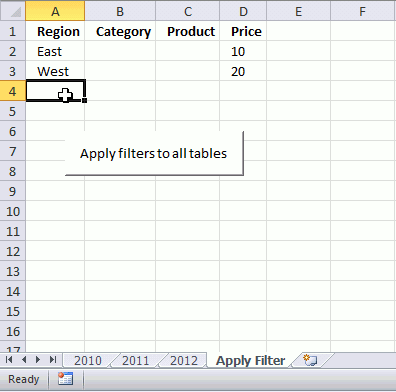It cycles through all excel tables in a workbook and applies the filter you have selected on a sheet.

### Apply filter to all excel tables and copy filtered values from all excel tables to a new sheet

So if you combine macro CopyFilteredTables and ApplyFilterToTable you can quickly apply multiple filters to all excel tables and then copy the filtered values from all excel tables to a new sheet. That saves you a lot of time.

Instructions

1. Select the criteria. You also need to specify the corresponding header, make sure you spell it right.
2. Run macro ApplyFilterToTable
3. A new sheet is created and populated with values from all filtered
```Sub ApplyCopy()</span>

ApplyFilterToTable
CopyFilteredTables

End Sub
```

### Clear all filters from all tables in active workbook

This macro clears all filters in all tables in active workbook.

```Sub ClearFiltersAllTables()
For Each WS In Worksheets
For Each tbl In WS.ListObjects
For ct = 1 To tbl.DataBodyRange.Columns.Count
tbl.Range.AutoFilter Field:=ct
Next ct
Next tbl
Next WS
End Sub
```

### Download excel *.xlsm file

Copy an excel table.xlsm